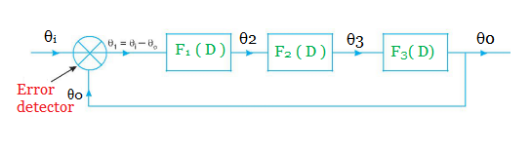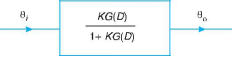## Friday, 8 July 2016

### Closed - Loop Transfer Function

Closed - Loop Transfer Function

The closed - loop transfer function is defined as the overall transfer function of the entire control system. It is a mathematical expression (algorithmdescribing the net result of the effects of a closed (feedbackloop. Consider a closed loop transfer function or feedback loop consisting of several elements as shown on Fig.,Fig Closed-loop transfer function.
Now, for the forward path element, we know that
where      K G (D ) = F1 (D ) X F2 (D ) X F3 (D)
On rearranging, we get
θo K G ( D )θi  K G ( D)θo
or             [1 + K G ( D )] θ= K G ( D)θi

The above expression shows the transfer function for the closed-loop control system. Thus the block diagram may be further simplified as shown in Fig., where the entire system is represented by a single block.Fig. Simplified closed-loop system.